Question

1.thuthuy

The wavelength is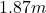Explanation:

Radio waves are part of the electromagnetic spectrum, any radiation of that electromagnetic spectrum has a speed of 3.00×10^{8}m/s in vacuum.

Therefore, the wavelength can be determined by the following equation: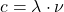(1)

Where c is the speed of light,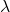is the wavelength and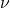is the frequency.

Then,can be isolated from equation 1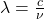(2)

Notice that it is necessary to express the frequency in units of Hz.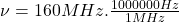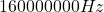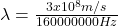But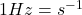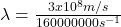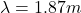Hence, the wavelength is